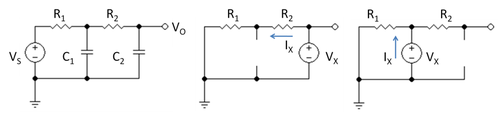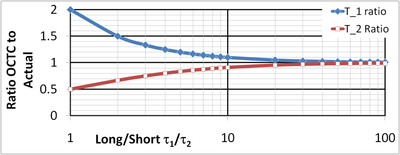# Open-circuit time constant method

﻿
Open-circuit time constant method

The open-circuit time constant method is an approximate analysis technique used in electronic circuit design to determine the corner frequency of complex circuits. It also is known as the zero-value time constant technique. The method provides a quick evaluation, and identifies the largest contributions to time constants as a guide to circuit improvements.

The basis of the method is the approximation that the corner frequency of the amplifier is determined by the term in the denominator of its transfer function that is linear in frequency. This approximation can be extremely inaccurate in some cases where a zero in the numerator is near in frequency.

The method also uses a simplified method for finding the term linear in frequency based upon summing the RC-products for each capacitor in the circuit, where the resistor R for a selected capacitor is the resistance found by inserting a test source at its site and setting all other capacitors to zero. Hence the name zero-value time constant technique.Figure 1: Simple RC circuit and auxiliary circuits to find time constants

## Example: Simple RC network

Figure 1 shows a simple RC low-pass filter. Its transfer function is found using Kirchhoff's current law as follows. At the output,$\frac {V_1 - V_O } {R_2} = j\omega C_2 V_O \ ,$

where V1 is the voltage at the top of capacitor C1. At the center node:$\frac {V_S-V_1}{R_1} = j \omega C_1 V_1 + \frac {V_1-V_O} {R_2} \ .$

Combining these relations the transfer function is found to be:$\frac {V_O} {V_S} = \frac {1} {1 + j \omega \left(C_2 (R_1+R_2) +C_1 R_1 \right) +(j \omega )^2 C_1 C_2 R_1 R_2 }$

The linear term in jω in this transfer function can be derived by the following method, which is an application of the open-circuit time constant method to this example.

1. Set the signal source to zero.
2. Select capacitor C2, replace it by a test voltage VX, and replace C1 by an open circuit. Then the resistance seen by the test voltage is found using the circuit in the middle panel of Figure 1 and is simply VX / IX = R1 + R2. Form the product C2 ( R1 + R2 ).
3. Select capacitor C1, replace it by a test voltage VX, and replace C2 by an open circuit. Then the resistance seen by the test voltage is found using the circuit in the right panel of Figure 1 and is simply VX / IX = R1. Form the product C1 R1.

In effect, it is as though each capacitor charges and discharges through the resistance found in the circuit when the other capacitor is an open circuit.

The open circuit time constant procedure provides the linear term in jω regardless of how complex the RC network becomes. For a complex circuit, the procedure consists of following the above rules, going through all the capacitors in the circuit. A more general derivation is found in Gray and Meyer. 

So far the result is general, but an approximation is introduced to make use of this result: the assumption is made that this linear term in jω determines the corner frequency of the circuit.

That assumption can be examined more closely using the example of Figure 1: suppose the time constants of this circuit are τ1 and τ2; that is:$\left( 1 + j \omega { \tau}_1) (1 +j \omega {\tau}_2 \right) = 1 + j \omega \left(C_2 (R_1+R_2) +C_1 R_1 \right) +(j \omega )^2 C_1 C_2 R_1 R_2$

Comparing the coefficients of the linear and quadratic terms in jω, there results:$\tau_1 + \tau_2 = C_2 (R_1+R_2) +C_1 R_1 \ ,$$\tau_1 \tau_2 = C_1 C_2 R_1 R_2 \ .$

One of the two time constants will be the longest; let it be τ1. Suppose for the moment that it is much larger than the other, τ1 >> τ2. In this case, the approximations hold that:$\tau_1 + \tau_2 \approx \tau_1 \ ,$

and$\tau_2 = \frac {\tau_1 \tau_2 } { \tau_1} \approx \frac {\tau_1 \tau_2 } { \tau_1 + \tau_2 } \ .$

In other words, substituting the RC-values:$\tau_1 \approx \hat { \tau_1} =\ \tau_1 + \tau_2 = C_2 (R_1+R_2) +C_1 R_1 \ ,$

and$\tau_2 \approx \hat { \tau_2} =\frac {\tau_1 \tau_2 } { \tau_1 + \tau_2 } = \frac {C_1 C_2 R_1 R_2} {C_2 (R_1+R_2) +C_1 R_1} \ ,$

where ( ^ ) denotes the approximate result. As an aside, notice that the circuit time constants both involve both capacitors; in other words, in general the circuit time constants are not decided by any single capacitor. Using these results, it is easy to explore how well the corner frequency (the 3dB frequency) is given by$f_{3dB} = \frac {1} {2 \pi \hat{ \tau_1 }} \ ,$Figure 2: Comparison of OCTC estimates with actual pole positions

as the parameters vary. Also, the exact transfer function can be compared with the approximate one, that is,$\frac {1} {(1+j \omega \tau_1)(1 + j \omega \tau_2)}\$$\$   with$\$$\ \frac {1} {(1+j \omega \hat {\tau_1 })(1 + j \omega \hat { \tau_2} )} \ .$

Of course agreement is good when the assumption τ1 >> τ2 is accurate.

Figure 2 illustrates the approximation. The x-axis is the ratio τ1 / τ2 on a logarithmic scale. An increase in this variable means the higher pole is further above the corner frequency. The y-axis is the ratio of the OCTC (open-circuit time constant) estimate to the true time constant. For the lowest pole use curve T_1; this curve refers to the corner frequency; and for the higher pole use curve T_2. The worst agreement is for τ1 = τ2. In this case τ^1 = 2 τ1 and the corner frequency is a factor of 2 too small. The higher pole is a factor of 2 too high (its time constant is half of the real value).

In all cases, the estimated corner frequency is closer than a factor of two from the real one, and always is conservative that is, lower than the real corner, so the actual circuit will behave better than predicted. However, the higher pole always is optimistic, that is, predicts the high pole at a higher frequency than really is the case. To use these estimates for step response predictions, which depend upon the ratio of the two pole frequencies (see article on pole splitting for an example), Figure 2 suggests a fairly large ratio of τ1 / τ2 is needed for accuracy because the errors in τ^1 and τ^2 reinforce each other in the ratio τ^1 / τ^2.

The open-circuit time constant method focuses upon the corner frequency alone, but as seen above, estimates for higher poles also are possible.

Application of the open-circuit time constant method to a number of single transistor amplifier stages can be found in Pittet and Kandaswamy.

## References and notes

1. ^ Marc T. Thompson (2006). Intuitive analog circuit design: a problem solving approach using design case studies. Oxford UK/ Amsterdam: Elsevier/Newnes. p. Chapter 7; p.161–167. ISBN 0-7506-7786-4.
2. ^ Paul R. Gray, Hurst P J Lewis S H & Meyer RG (2001). Analysis and design of analog integrated circuits (Fourth Edition ed.). New York: Wiley. p. §7.3.2 pp. 517–520. ISBN 0-471-32168-0.
3. ^ Andre Pittet & A. Kandaswamy (2005). Analog electronics. New Delhi: Prentice-Hall of India. p. Chapter 4; pp. 155–166. ISBN 8120327845.

Wikimedia Foundation. 2010.

### Look at other dictionaries:

• Time constant — In physics and engineering, the time constant usually denoted by the Greek letter au , (tau), characterizes the frequency response of a first order, linear time invariant (LTI) system. Examples include electrical RC circuits and RL circuits. It… …   Wikipedia

• Constant voltage method — is a type of MPPT algorithm. This method makes use of the fact that the ratio of maximum power point voltage and the open circuit voltage is 0.76. It is the simplest MPPT control method. Algorithm The operating point of the PV array is… …   Wikipedia

• Time-domain reflectometer — A time domain reflectometer (TDR) is an electronic instrument used to characterize and locate faults in metallic cables (for example, twisted wire pairs, coaxial cables). The equivalent device for optical fiber is an optical time domain… …   Wikipedia

• Transformer types — Circuit symbols Transformer with two windings and iron core. Step down or step up transformer. The symbol shows which winding has more turns, but not usually the …   Wikipedia

• Scuba set — A scuba diver in usual sport diving gear A scuba set is an independent breathing set that provides a scuba diver with the breathing gas necessary to breathe underwater during scuba diving. It is much used for sport diving and some sorts of work… …   Wikipedia

• Glossary of fuel cell terms — The Glossary of fuel cell terms lists the definitions of many terms used within the fuel cell industry. The terms in this glossary may be used by fuel cell industry associations, in education material and fuel cell codes and standards to name but …   Wikipedia

• PID controller — A block diagram of a PID controller A proportional–integral–derivative controller (PID controller) is a generic control loop feedback mechanism (controller) widely used in industrial control systems – a PID is the most commonly used feedback… …   Wikipedia

• Network analysis (electrical circuits) — Linear Network Analysis Elements …   Wikipedia

• Charlieplexing — is a technique proposed in early 1995 by Charlie Allen at Maxim Integrated Products for driving a multiplexed display in which relatively few I/O pins on a microcontroller are used to drive an array of LEDs. The method utilizes the tri state… …   Wikipedia

• Operational amplifier — A Signetics μa741 operational amplifier, one of the most successful op amps. An operational amplifier ( op amp ) is a DC coupled high gain electronic voltage amplifier with a differential input and, usually, a single ended output. An op amp… …   Wikipedia